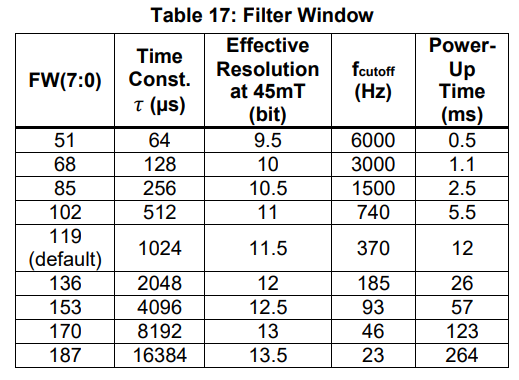# Filter Window definition by Maximum RPM

How do i define and configure the FW Filter Window parameter by material measure and by maximum RPM speed?

The filter window parameter does not depend at all on the maximum RPM. We have a constant latency (8 us for most products) regardless of RPM as long as the RPM is constant.

That last part is important and hints at what really matters - the rate of acceleration when spinning up / down. The worst case latency while accelerating (or decelerating which is treated here as a negative acceleration) can be calculated by L = a * Tau^2 where
L is lag (in degrees or radians)
a is max accleleration
and Tau is the time constant of the particular filter window you are using (can be found in the datasheet)

As an example, let’s take the MA732 with a filter window set to 136.By this chart (found under the ‘REGISTER SETTINGS’ section of the datasheet) we can see that Tau = 2048 us. If we then assume a motor with a max acceleration of 100 rad / s^2, we find a max lag by:

L = 100 rad/s^2 * (2048 x 10^-6 s)^2 = 4.19 * 10^-4 radians

4.19 * 10^-4 radians * 360 deg / 2 * pi radians = 0.02 degrees lag